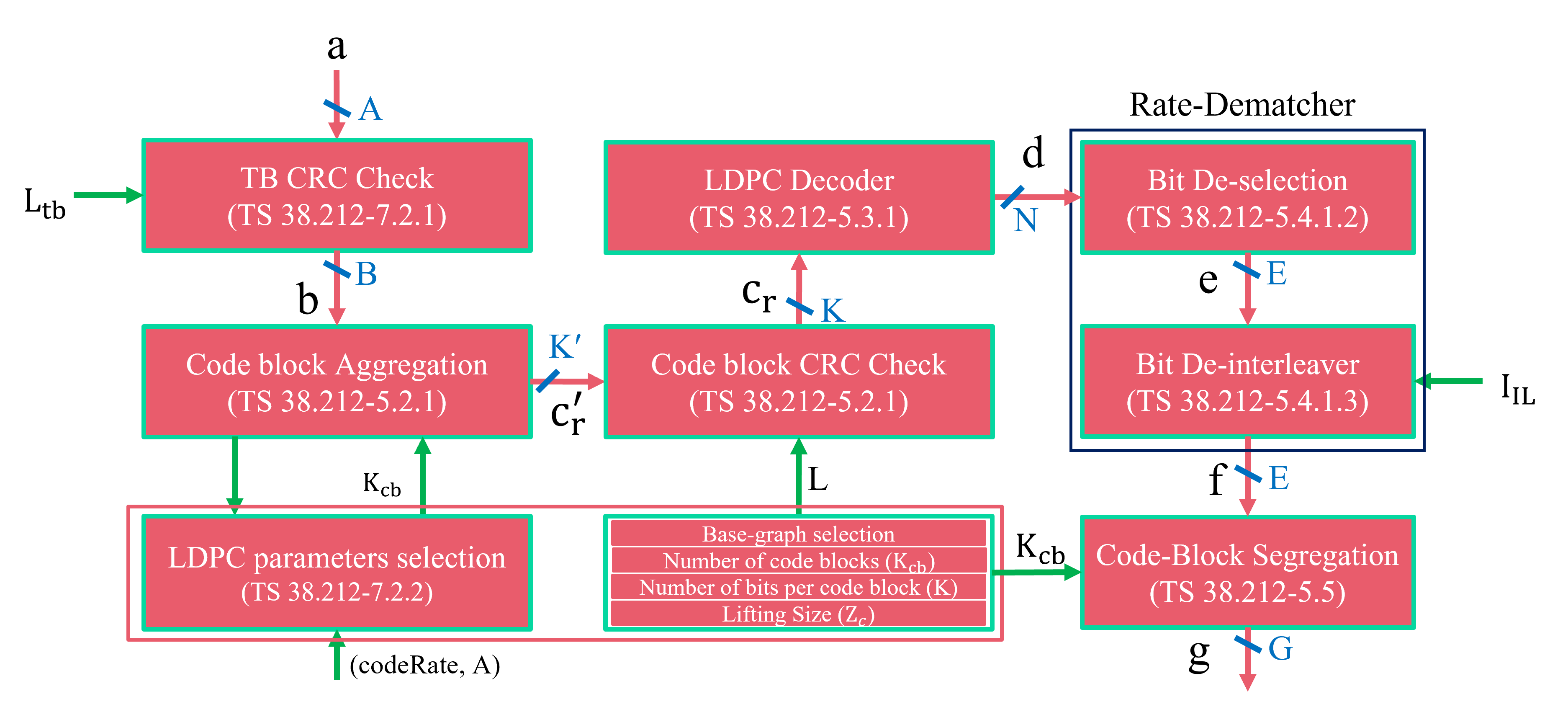# PDSCH: Upper Physical layer Chain Decoder

This module implements the physical downlink shared channel.The following code block illustrates how to initialize and invoke PDSCH Upper Phy Decoder

```numSymbols         = 13
numRB              = 25
mcsIndex           = 2
numlayers          = 
scalingField       = '00'
dmrsREs            = 3
numTBs             = 1
mcsTable           = 'pdschTable2'

#LLRs  = An array of estimated likelihood ratios (LLRs)of size (...,numTargetBits)

DecodedBits               = pdschDecoderUpperPhyChain()
```

The details of the input-output interface are provided in following sub-section.

class toolkit5G.PhysicalChannels.PDSCHDecoderUpperPhy(numTBs, mcsIndex, symbolsPerSlot, numRB, numLayers, scalingField, additionalOverhead=0, dmrsREs=3, enableLBRM=[False, False], pdschTable='pdschTable1', rvid=[0, 0], verbose=True)[source]

This class performs Receiver side of Upper Physical Layer Processing of Physical Downlink Shared Channel.

Parameters:
• numTBs (int) – The Physical layer processes either 1 or 2 Transport Blocks for each resource allocation. So it represents number of Transport Blocks to be processed.

• mcsIndex (int or np.ndarray) – Refers to Modulation and Coding Scheme (MCS) Index. It takes Either a Scalar or a numpy array based on the argument passed as pdschTable

-if `pdschTable` is passed as none, mcsIndex expects a numpy array of [modulation order, code Rate]

-if `pdschTable` argument is passed , Based on it the following Lower Bound and Upper Bound for mcs Index is followed:

Table

LowerBound

Upperbound

PDSCH Table 1

0

28

PDSCH Table 2

0

27

PDSCH Table 3

0

28

PDSCH Table 4

0

26

PUSCH Table 1

0

27

PUSCH Table 2

0

27

CQI Table 1

1

15

CQI Table 2

1

15

CQI Table 3

1

15

CQI Table 4

1

15

• symbolsPerSlot (int) – Number of symbols allocated per Slot. It takes a scalar or numpy integer. It can take values between 1 and 14.

• numRB (int) – Number of Physical Resource Blocks allocated. It takes a positive scalar or numpy integer.

• numlayers (numpy array) – Number of MIMO layers (the number of layers is equal to the number of allocated DMRS Ports). It takes numpy array containing integers of length 2. If the number of Transport Blocks processed is 2, then it can take values between 1 and 8. Otherwise, it can take value between 1 and 4.

• scalingField (str) – Transport Block Scaling Field. It accepts a string. Valid Strings are ‘00’, ‘01’, ‘10’.

• rvID; numpy array of integer(s) – Redundancy Version ID which selects the starting position for the extracting the bits from the buffer for the corresponding Transport Block processing

• additionalOverhead (int) – Additional Overheads that results in reduced number of available Resource Elements for data transfer. It takes a scalar or numpy integer. Valid values are 0, 6, 12 or 18.

• dmrsREs (int) – Number of Resource Elements per Resource Block allocated to Demodulation Reference Signal(DMRS). By default, it set to 3

• pdschTable (str) – The Lookup table to be used for the selection of Modulation Order and code Rate It can take the strings ‘pdschTable1’, ‘pdschTable2’, ‘pdschTable3’, ‘pdschTable4’, ‘puschTable1’, ‘puschTable2’ ,’cqiTable1’, ‘cqiTable2’, ‘cqiTable3’, ‘cqiTable4’

• enableLBRM (numpy array of bool) – Enable / Disable Limited Buffer Rate Matching. The concept of LBRM to help reduce the requirement for buffering large code blocks, thus minimalizing the memory required. The length of the array is 2 based on the number of transport block that can be processed in the downlink direction. By default, it set to [‘False’, ‘False’]

• verbose (bool) – By enabling verbose, it displays information about the progress in the execution of the modules. Such as size of the input/output vectors inputed to the modules and parameters being passed. By default, set to ‘True’.

Input:

symbolLLRs ((…, numberTargetBits), float32) – An array of estimated likelihood ratios (LLRs).

Returns:

codeword – Either a numpy array or list of numpy array based on the number of TBs. The arrays are the Bits estimated from the given LLR The dimensions of the array are (numBatches,numBSs,numTargetBits)

Return type:

[`numTBs`,], list

Attributes:
• Accessed using``<PDSCH Decoder Upper Phy object>.<attribute name>

• modOrder (int) – Modulation order

• codeRate (float) – Code Rate

• numCBs (int) – Number of Code Blocks

• numTargetBits (int) – Number of target Bits at the output of Rate matcher

• k_ldpc and/or k_ldpc2 (int) – Number of Information Bits. k_ldpc2 may or may not exist based on the existence of numTBs

• n and/or n2 (int) – Number of Size of LDPC codeword after internal truncation. n2 may or may not exist based on the existence of numTBs

• n_ldpc and/or n_ldpc2 (int) – Size of the codeword generated by the LDPC encoder before truncation. n_ldpc2 may or may not exist based on the existence of numTBs

• liftingfactor and/or liftingFactor2 (int) – Defines the lifting factor to be used by the LDPC codec for the given TB size and code-rate.liftingfactor2 may or may not exist based on the existence of numTBs

• fillerIndices and/or fillerIndices2 (np.ndarray) – Indices where filler Bits were inserted

Note

It is to be taken into consideration that the Upper Phy Decoder arguments should be consistent with the corresponding transmitter side Upper Phy and Lower Phy or else the implementation may collapse